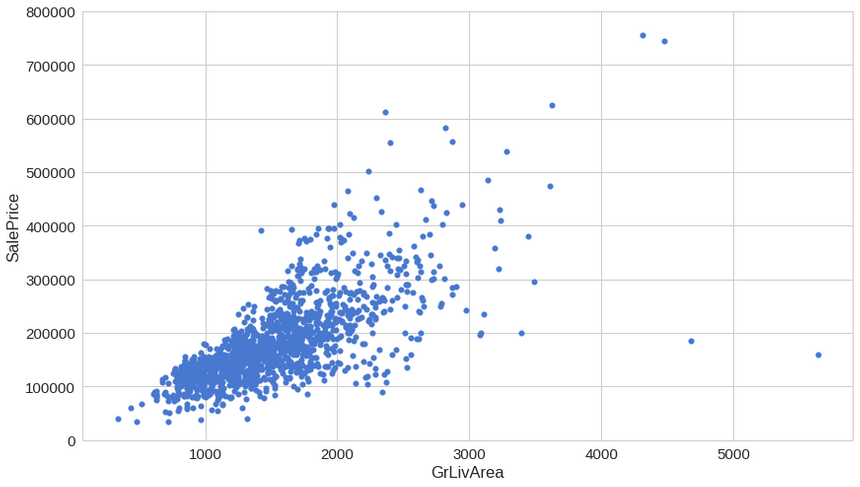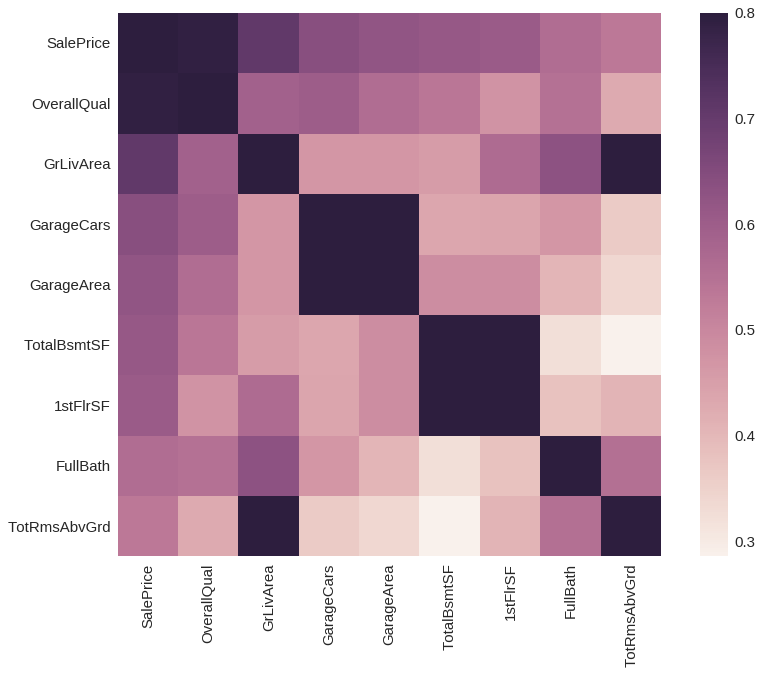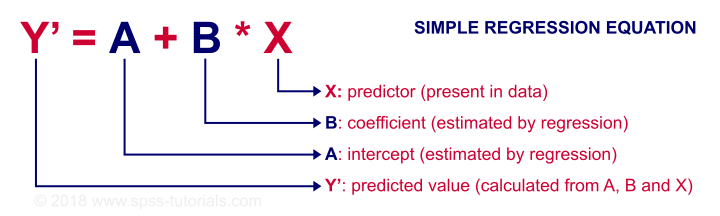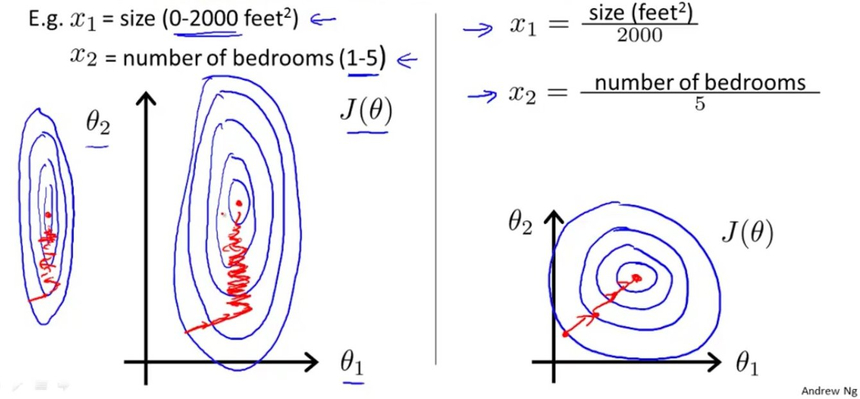# Curiousily

## Predicting House Prices with Linear Regression | Machine Learning from Scratch (Part II)

Machine Learning, Statistics, Linear Regression4 min read

Share

TL;DR Use a test-driven approach to build a Linear Regression model using Python from scratch. You will use your trained model to predict house sale prices and extend it to a multivariate Linear Regression.

I know that you’ve always dreamed of dominating the housing market. Until now, that was impossible. But with this limited offer you can… got a bit sidetracked there.

Let’s start building our model with Python, but this time we will use it on a more realistic dataset.

Complete source code notebook on Google Colaboratory

# The Data

Our data comes from a Kaggle competition named “House Prices: Advanced Regression Techniques”. It contains 1460 training data points and 80 features that might help us predict the selling price of a house.

Let’s load the Kaggle dataset into a Pandas data frame:

1df_train = pd.read_csv('house_prices_train.csv')

# Exploration  —  getting a feel for our data

We’re going to predict the SalePrice column (\$ USD), let’s start with it:

1count      1460.0000002mean     180921.1958903std       79442.5028834min       34900.000000525%      129975.000000650%      163000.000000775%      214000.0000008max      755000.0000009Name: SalePrice, dtype: float64Most of the density lies between 100k and 250k, but there appears to be a lot of outliers on the pricier side.

Next, let’s have a look at the greater living area (square feet) against the sale price:You might’ve expected that larger living area should mean a higher price. This chart shows you’re generally correct. But what are those 2–3 “cheap” houses offering huge living area?

One column you might not think about exploring is the TotalBsmtSF  —  Total square feet of the basement area, but let’s do it anyway:Intriguing, isn’t it? The basement area seems like it might have a lot of predictive power for our model.

Ok, last one. Let’s look at “OverallQual” — overall material and finish quality. Of course, this one seems like a much more subjective feature, so it might provide a bit different perspective on the sale price.Everything seems fine for this one, except that when you look to the right things start getting much more nuanced. Will that “confuse” our model?

Let’s have a more general view on the top 8 correlated features with the sale price:Surprised? All the features we discussed so far appear to be present. Its almost like we knew them from the start…

## Do we have missing data?

We still haven’t discussed ways to “handle” missing data, so we’ll handle them like a boss — just not use those features:

Row countPercentage
PoolQC14530.995205
MiscFeature14060.963014
Alley13690.937671
Fence11790.807534
FireplaceQu6900.472603
LotFrontage2590.177397
GarageCond810.055479
GarageType810.055479
GarageYrBlt810.055479
GarageFinish810.055479
GarageQual810.055479
BsmtExposure380.026027
BsmtFinType2380.026027
BsmtFinType1370.025342
BsmtCond370.025342

Yes, we’re not going to use any of those.

# Predicting the sale price

Now that we have some feel of the data we’re playing with we can start our plan of attack — how to predict the sale price for a given house?

## Using Linear Regressionsource: http://mybooksucks.com

Linear regression models assume that the relationship between a dependent continuous variable Y and one or more explanatory (independent) variables X is linear (that is, a straight line).

It’s used to predict values within a continuous range (e.g. sales, price) rather than trying to classify them into categories (e.g. cat, dog). Linear regression models can be divided into two main types:

### Simple Linear Regression

Simple linear regression uses a traditional slope-intercept form, where a and b are the coefficients that we try to “learn” and produce the most accurate predictions. $X$ represents our input data and $Y$ is our prediction.

$Y = bX + a$source: https://spss-tutorials.com

### Multivariable Regression

A more complex, multi-variable linear equation might look like this, where w represents the coefficients or weights, our model will try to learn.

$Y(x_1,x_2,x_3) = w_1 x_1 + w_2 x_2 + w_3 x_3 + w_0$

The variables $x_1, x_2, x_3$ represent the attributes or distinct pieces of information, we have about each observation.

## Loss function

Given our Simple Linear Regression equation:

$Y = bX + a$

We can use the following cost function to find the coefficients/parameters for our model:

### Mean Squared Error (MSE) Cost Function

The MSE is defined as:

$MSE = J(W) = \frac{1}{m} \sum_{i=1}^{m} (y^{(i)} - h_w(x^{(i)}))^2$

where

$h_w(x) = g(w^Tx)$

The MSE measures how much the average model predictions vary from the correct values. The number is higher when the model is performing “bad” on our training data.

The first derivative of MSE is given by:

$MSE' = J'(W) = \frac{2}{m} \sum_{i=1}^{m} (h_w(x^{(i)}) - y^{(i)})$

### One Half Mean Squared Error (OHMSE)

We will apply a small modification to the MSE — multiply by $\frac{1}{2}$ so when we take the derivative, the 2s cancel out:

$OHMSE = J(W) = \frac{1}{2m} \sum_{i=1}^{m} (y^{(i)} - h_w(x^{(i)}))^2$

The first derivative of OHMSE is given by:

$OHMSE' = J'(W) = \frac{1}{m} \sum_{i=1}^{m} (h_w(x^{(i)}) - y^{(i)})$

Let’s implement it in Python (yes, we’re going TDD style!)

1class TestLoss(unittest.TestCase):23  def test_zero_h_zero_y(self):4    self.assertAlmostEqual(loss(h=np.array(), y=np.array()), 0)56  def test_one_h_zero_y(self):7    self.assertAlmostEqual(loss(h=np.array(), y=np.array()), 0.5)89  def test_two_h_zero_y(self):10    self.assertAlmostEqual(loss(h=np.array(), y=np.array()), 2)1112  def test_zero_h_one_y(self):13    self.assertAlmostEqual(loss(h=np.array(), y=np.array()), 0.5)1415  def test_zero_h_two_y(self):16    self.assertAlmostEqual(loss(h=np.array(), y=np.array()), 2)

Now that we have the tests ready, we can implement the loss function:

1def loss(h, y):2  sq_error = (h - y)**23  n = len(y)4  return 1.0 / (2*n) * sq_error.sum()
1run_tests()

time for the results:

1.....23--------------------------------------------------------------4Ran 5 tests in 0.007s5OK

## Data preprocessing

We will do a little preprocessing to our data using the following formula (standardization):

$x'= \frac{x - \mu}{\sigma}$

where $\mu$ is the population mean and $\sigma$ is the standard deviation.

But why? Why would we want to do that? The following chart might help you out:source: Andrew Ng

What the doodle shows us that our old friend — the gradient descent algorithm, might converge (find good parameters) faster when our training data is scaled. Shall we?

1x = df_train['GrLivArea']2y = df_train['SalePrice']34x = (x - x.mean()) / x.std()5x = np.c_[np.ones(x.shape), x]

We will only use the greater living area feature for our first model.

## Implementing Linear Regression

First, our tests:

1class TestLinearRegression(unittest.TestCase):23    def test_find_coefficients(self):4      clf = LinearRegression()5      clf.fit(x, y, n_iter=2000, lr=0.01)6      np.testing.assert_array_almost_equal(7          clf._W,8          np.array([180921.19555322,  56294.90199925])9      )

Without further ado, the simple linear regression implementation:

1class LinearRegression:23  def predict(self, X):4    return np.dot(X, self._W)56  def _gradient_descent_step(self, X, targets, lr):78    predictions = self.predict(X)910    error = predictions - targets11    gradient = np.dot(X.T,  error) / len(X)1213    self._W -= lr * gradient1415  def fit(self, X, y, n_iter=100000, lr=0.01):1617    self._W = np.zeros(X.shape)1819    for i in range(n_iter):20        self._gradient_descent_step(x, y, lr)2122    return self

You might find our Linear Regression implementation simpler compared to the one presented for the Logistic Regression. Note that the use of the Gradient Descent algorithm is pretty much the same. Interesting, isn’t it? A single algorithm can build two different types of models. Would we be able to use it for more?

1run_tests()
1......23-------------------------------------- Ran 6 tests in 1.094s4OK

## Predicting the sale price with our first model

Let’s use our freshly created model to begin our housing market domination:

1clf = LinearRegression()2clf.fit(x, y, n_iter=2000, lr=0.01)

So how did the training go?Our cost, at the last iteration, has a value of:

11569921604.8332634

Can we do better?

## Multivariable Linear Regression

Let’s use more of the available data to build a Multivariable Linear Regression model and see whether or not that will improve our OHMSE error. Let’s not forget that scaling too:

1x = df_train[['OverallQual', 'GrLivArea', 'GarageCars']]23x = (x - x.mean()) / x.std()4x = np.c_[np.ones(x.shape), x]

### Implementing Multivariable Linear Regression

This space is intentionally left (kinda) blank

### Using Multivariable Linear Regression

Now that the implementation of our new model is done, we can use it. Done!?

You see, young padawan, the magical world of software development has those mythical creates called abstractions. While it might be extraordinarily hard to get them right, they might reduce the complexity of the code you write by (pretty much) a ton.

You just used one such abstraction — called vectorization. Essentially, that allowed us to build a Multivariable Linear Regression model without the need to loop over all features in our dataset. Neat, right?

Our client interface stays the same, too:

1clf = LinearRegression()2clf.fit(x, y, n_iter=2000, lr=0.01)

And the results:1822817042.8437098

The loss at the last iteration is nearly 2 times smaller. Does this mean that our model is better now?

Complete source code notebook on Google Colaboratory

# Conclusion

Nice! You just implemented a Linear Regression model and not the simple/crappy kind.

One thing you might want to try is to predict house sale prices on the testing dataset from Kaggle. Is this model any good on it?

In the next part, you’re going to implement a Decision Tree model from scratch!

Share

## Want to be a Machine Learning expert?

Join the weekly newsletter on Data Science, Deep Learning and Machine Learning in your inbox, curated by me! Chosen by 10,000+ Machine Learning practitioners. (There might be some exclusive content, too!)

You'll never get spam from me

© 2020 Curiousily by Venelin Valkov